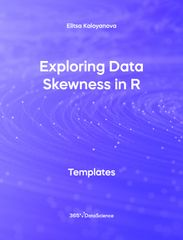# Templates

Boost your programming skills with free Python, SQL, R, and Excel templates and create top-notch projects for your portfolio.

Save hours of browsing through the Internet and accelerate your workflow with code-ready templates for your programming projects. Personally crafted by 365 Data Science instructors, these templates bring a surprising efficiency level to your work process.Templates excel

## Side by Side Bar Chart in Excel Template

This Side by Side Bar Chart in Excel uses a cross-table for the construction of a side-by-side bar chart. Some other related topics you might be interested to explore are Cross Bar chart in Excel, Bar chart in Excel, Pie chart in Excel, Histogram in Excel, Frequency Distribution Table for Numerical Variables You can now download the Excel template for free. Cross Table in Excel is among the topics covered in detail in the 365 Data Science program.Templates excel

## Histogram in Excel Template

This Histogram in Excel includes a sample dataset, a frequency distribution table constructed from this dataset, and 2 histograms visualizing the data - one representing frequency and a second one representing relative frequency. Some other related topics you might be interested to explore are Pie Chart in Excel, Line Chart in Excel , Bar and Line Chart in Excel and Stacked Area Chart in Excel. You can now download the Excel template for free. Histogram in Excel is among the topics covered in detail in the 365 Data Science program.Templates excel

## Frequency Distribution Table for Numerical Variables in Excel Template

This Frequency Distribution Table for Numerical Variables in Excel template demonstrates the typical construction of a frequency distribution table. It shows how the data can be divided into intervals, how the count of items in a given interval is performed and how relative frequencies are calculated. Some other related topics you might be interested to explore are Pie Chart in Excel, Line Chart in Excel , Bar and Line Chart in Excel and Stacked Area Chart in Excel. You can now download the Excel template for free. Frequency Distribution Table for Numerical Variables in Excel is among the topics covered in detail in the 365 Data Science program.Templates excel

## Cross Table in Excel Template

This Cross Table in Excel shows how a typical cross-table can be constructed. The rows of the table represent the type of investment while the investors are placed as columns. Each cell, therefore, shows how much each person invested and where. Some other related topics you might be interested to explore are Side-by-side bar chart in Excel, Bar chart in Excel, Pie chart in Excel, Histogram in Excel, Frequency Distribution Table for Numerical Variables. You can now download the Excel template for free. Cross Table in Excel is among the topics covered in detail in the 365 Data Science program.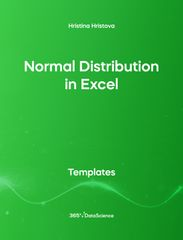Templates excel

## Normal Distribution in Excel Template

This Normal Distribution in Excel template demonstrates that the sum of 2 randomly thrown dice is normally distributed. Open the .xlsx file with Microsoft Excel. Study the structure of the file and experiment with different values. Some other related topics you might be interested to explore are Positive Skew in Excel, Zero Skew in Excel, Negative Skew in Excel, Uniform Distribution in Excel, Standard Normal Distribution in Excel You can now download the Excel template for free. Normal Distribution in Excel is among the topics covered in detail in the 365 Data Science programTemplates excel

## Scatter Plot in Excel Template

This Scatter Plot in Excel Template displays the relationship between the area and price of a California real estate data set, allowing us to see if any connection exists between the two variables. Some other related topics you might be interested to explore are Pie Chart in Excel, Line Chart in Excel , Bar and Line Chart in Excel and Stacked Area Chart in Excel. You can now download the Excel template for free. Scatter Plot in Excel is among the topics covered in detail in the 365 Data Science program.Templates excel

## Pareto Diagram in Excel Template

This Pareto Diagram in Excel shows how visualize categorical data, by representing the number of cars per brand a car shop has sold. Some other related topics you might be interested to explore are Pie Chart in Excel, Line Chart in Excel , Bar and Line Chart in Excel and Stacked Area Chart in Excel. You can now download the Excel template for free. Pareto Diagram in Excel is among the topics covered in detail in the 365 Data Science program.Templates excel

## Bar and Line Chart in Excel

This Bar and Line Chart in Excel Template uses a dual y-axis and shows the number of participants of a survey using a bar chart and the percentage among them using the Python programming language using a line chart. Some other related topics you might be interested in exploring are Scatter Plot in Excel, Pie Chart in Excel, Line Chart in Excel, and Stacked Area Chart in Excel. You can now download the Excel template for free. Bar and Line Chart in Excel is among the topics covered in detail in the 365 Data Science program.Templates r

## While Loops in R Template

The While Loops in R shows how to create a while loop which sums up the numbers from 1 to n. A while loop operates as long as, or while its condition evaluates to TRUE. In contrast to a for loop which iterates over a sequence of number, the while loop evaluates whether a condition holds or not. Some other related topics you might be interested in exploring are For Loops in R and If Else Else If Statements in R. You can now download the R template for free. Calculating Data Variance in R is among the topics covered in detail in the 365 Data Science program.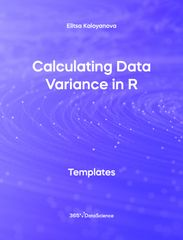Templates r

## Calculating Data Variance in R Template

The Calculating Data variance in R shows how variance measures the dispersion of a set of data points around their mean value. Some other related topics you might be interested are Calculating Standard Deviation in R, Calculating Data Mean in R, Calculating Standard Deviation of Data in R, Exploring Data Skewness in R You can now download the R template for free. Calculating Data Variance in R is among the topics covered in detail in the 365 Data Science program.Templates r

## If Else Else If Statements in R Template

The If Else Else IF Statements in R template shows whether a certain condition is met and can perform distinct actions provided the condition is met, and provided it is not. Some other related topics you might be interested in exploring are While loops in R and For Loops in R You can now download the R template for free. If Else Else If Statements in R is among the topics covered in detail in the 365 Data Science program.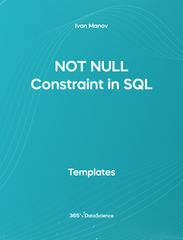Templates SQL

## Not Null Constraint in SQL Template

The Not Null Constraint in SQL template shows you how to assign a PRIMARY KEY constraint, specifying that the values of the constrained email must uniquely identify each row. Download and unzip the .zip file in a new folder. Inside the folder you will find a .sql file. Load it into MySQL Workbench. Some other related topics you might be interested are Primary Key Constraint in SQL, Unique Constraint in SQL and Default Constraint in SQL . You can now download the SQL template for free. Not Null Constraint in SQL is among the topics covered in detail in the 365 Data Science program.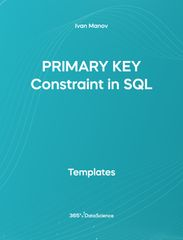Templates SQL

## Primary Key Constraint in SQL Template

The Primary Key Constraint in SQL template shows you how to assign a Primary Key constraint, specifying that the values of the constrained email must uniquely identify each row. Download and unzip the .zip file in a new folder. Inside the folder you will find a .sql file. Load it into MySQL Workbench. Some other related topics you might be interested are Not Null Constraint, Unique Constraint and Default Constraint. You can now download the SQL template for free. Primary Key Constraint in SQL is among the topics covered in detail in the 365 Data Science program.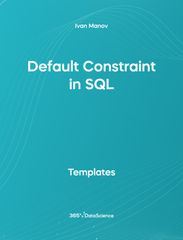Templates SQL

## Default Constraint in SQL Template

The Default Constraint in SQL template shows you how to assign a default value to a column in a table. If no other value is specified it will be added to all new records. Download and unzip the .zip file in a new folder. Inside the folder you will find a .sql file. Load it into MySQL Workbench. Some other related topics you might be interested are Primary Key Constraint in SQL, Not Null Constraint, and Unique Constraint. You can now download the SQL template for free. Default Constraint in SQL is among the topics covered in detail in the 365 Data Science program.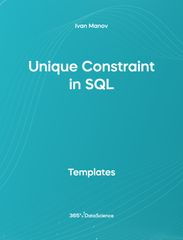Templates SQL

## Unique Constraint in SQL Template

The Unique Constraint in SQL template shows you how to assign a UNIQUE KEY constraint to a table, used to avoid any duplicates and ensure unique values in the column. Download and unzip the .zip file in a new folder. Inside the folder you will find a .sql file. Load it into MySQL Workbench. Some other related topics you might be interested are Primary Key Constraint in SQL, Not Null Constraint, and Default Constraint. You can now download the SQL template for free. Unique Constraint in SQL is among the topics covered in detail in the 365 Data Science program.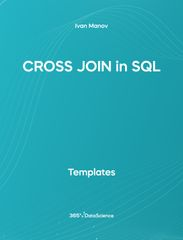Templates SQL

## Cross Join in SQL Template

The Cross Join in SQL template shows you how to take the values from a certain table and connects them with all the values from the tables we want to join it with. Download and unzip the .zip file in a new folder. Inside the folder you will find a .sql file. Load it into MySQL Workbench. Some other related topics you might be interested are Inner JOIN in SQL, Left JOIN in SQL, and Right JOIN in SQL. You can now download the SQL template for free. Cross JOIN in SQL is among the topics covered in detail in the 365 Data Science program.Templates SQL

## Right Join in SQL Template

The Right Join in SQL template shows you how to create a new instance of a table combining rows that have matching values from two tables plus all values from the right table that match no values from the left one. Download and unzip the .zip file in a new folder. Inside the folder you will find a .sql file. Load it into MySQL Workbench. Some other related topics you might be interested are Inner JOIN in SQL, Left JOIN in SQL, and Cross JOIN in SQL. You can now download the SQL template for free. Right JOIN in SQL is among the topics covered in detail in the 365 Data Science program.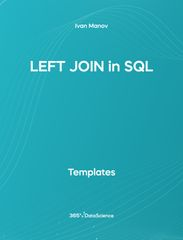Templates SQL

## Left Join in SQL Template

The Left JOIN in SQL template shows you how to create a new instance of a table combining rows that have matching values from two tables plus all values from the left table that match no values from the right one. Download and unzip the .zip file in a new folder. Inside the folder you will find a .sql file. Load it into MySQL Workbench. Some other related topics you might be interested are Inner JOIN in SQL, Right JOIN in SQL, and Cross JOIN in SQL. You can now download the SQL template for free. Left JOIN in SQL is among the topics covered in detail in the 365 Data Science program.Templates r

## Scatter Plot with ggplot2 in R Template

The Scatter Plot with ggplot2 in R includes a color scheme from the Wes Anderson movie palette. The chart displays the relationship between the price and area of houses from a property data set. A scatter plot is one of the most widely used charts in statistics and data analytics. It shows a collection of data points across two axes, signifying two categories of the data we wish to compare. Some other related topics you might be interested are Bar chart with ggplot2 in R, Histogram with ggplot2 in R, Regression Scatter Plot with ggplot2 in R, and Line Chart with ggplot2 in R. You can now download the R template for free.